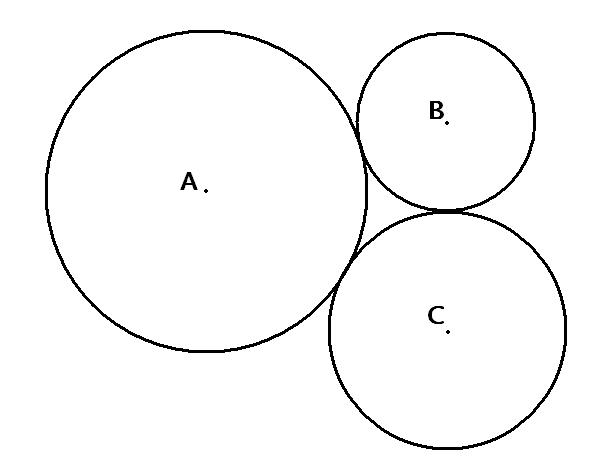# A geometry problem by Riel Diala

Geometry Level 1The length of line segment AB is 24 units.
The length of line segment AC is 25 units.
The length of line segment BC is 19 units.
QUESTION: What is the radius of circle A?

NOTE: Figure may or may NOT be drawn to scale, since this math problem can be solved without a picture.

×